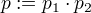## Probabilities

A probability [that an event occurs] is a number between 0 and 1.

### Logical Or

If the probabilityfor an event occuring is given and the probabilityfor another event (disjunct from the first) occuring is given, then the probability p of either (or both) occuring is:### Logical And

If the probabilityfor an event occuring is given and the probabilityfor another event (disjunct from the first) occuring is given, then the probability p of both occuring is:If the probabilityof an event is known, and this event can only happen after some other event with probabilityhappened, then the probability p of the event 1 happening is:(this is a special case for independent events)